# Algebra 1 : How to graph a function

## Example Questions

### Example Question #1 : Introduction To Functions

Which graph depicts a function?

Explanation:

A function may only have one y-value for each x-value.

The vertical line test can be used to identify the function. If at any point on the graph, a straight vertical line intersects the curve at more than one point, the curve is not a function.

### Example Question #1 : How To Graph A Function

The graph below is the graph of a piece-wise function in some interval.  Identify, in interval notation, the decreasing interval.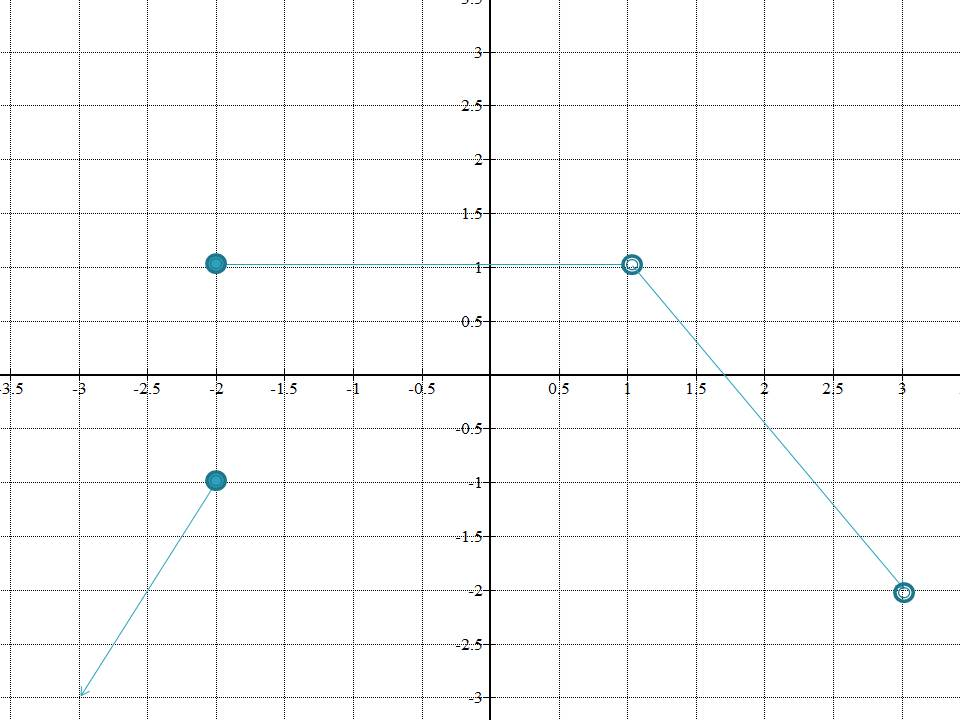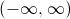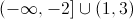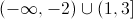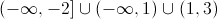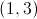Explanation:

As is clear from the graph, in the interval between(included) to, theis constant atand then from(not included) to(not included), theis a decreasing function.

### Example Question #3 : Graphing Polynomial Functions

Which equation best represents the following graph?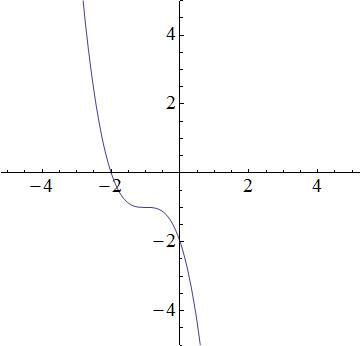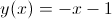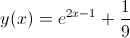None of these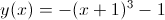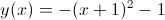Explanation:

We have the following answer choices.

1.2.3.4.The first equation is a cubic function, which produces a function similar to the graph. The second equation is quadratic and thus, a parabola. The graph does not look like a prabola, so the 2nd equation will be incorrect. The third equation describes a line, but the graph is not linear; the third equation is incorrect. The fourth equation is incorrect because it is an exponential, and the graph is not an exponential. So that leaves the first equation as the best possible choice.